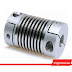As many couplings act as spring or use the concept of springs behind their construction and operation, spring stiffness is the factor that affects in selection of couplings.
When a coupling experiences a load in a direction that places it in compression or tension, its spring stiffness comes into effect. This can be described by using Hooke's law. This law relates the force and displacement of the spring using its spring constant. This value is different for different materials.
However, Hooke's law does not work for every force and displacement situations because materials are not always perfectly elastic in every load condition. Neverthless, it works well for the kinds of conditions most couplings operate in.
If the coupling is expected to find use in situations that involve tension or compression, then the relationship governing their expansion and contraction is F=kX
where, X = displacement of the spring or the coupling
k = spring constant
and F = Force applied.
Rearranging above equation gives, X=F/k
This is useful in systems using couplings because by knowing the spring constant and force, it is simple to find out the displacement the coupling will experience. Because this is a linear relationship to stretch a coupling twice as far required twice as force and so on.
When Engineer's select coupling, Hooke's law is used to check quickly if the amount of displacement is acceptable and can help to determine if a certain coupling is right for the system or not. While not a perfect description of spring behavior, for must use cases, this calculation provides a simple check that can prevent troubles in the future.
Effect of Spring Stiffness in Coupling selectionReviewed by Admin on 00:10 Rating: 5